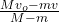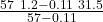Question

A 57 kg ice skater moving to the right with a velocity of 1.2 m/s throws a 0.11 kg snowball to the right with a velocity of 31.5 m/s relative to the ground. What is the velocity of the ice skater after throwing the snowball? Disregard the friction between the skates and the ice.

1.binhan

v_f = 1.14 m / s

Explanation:

This is an exercise of conservation of momentum, let’s define a system formed by the skater and the snowball, therefore the forces during the launch are internal and the moment is conserved.

Initial instant. Before throwing the ball

p₀ = M v₀

Final moment. After throwing the snowball

p_f = (M-m) v_f + m v

the moment is preserved

p₀ = p_f

M v₀ = (M-m) v_f + m v

v_f =let’s calculate

v_f =v_f = 64.936 / 56.89

v_f = 1.14 m / s